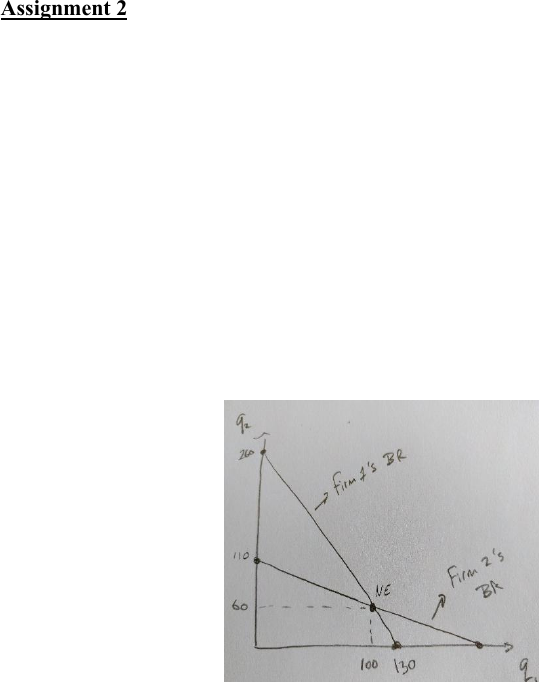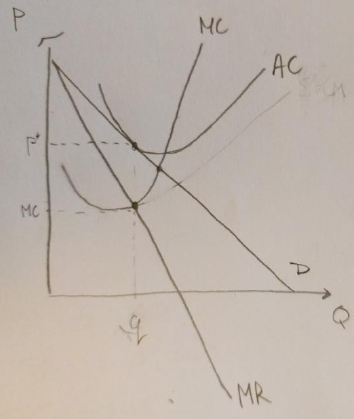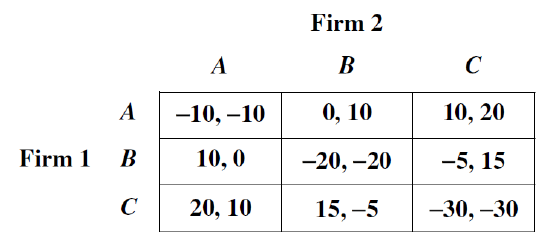# COMM 295 Study Guide - Quiz Guide: Nash Equilibrium, Cournot Competition, Inverse Demand Function

171 views14 pages1
Commerce 295/FRE 295
Fall 2015
Assignment 2
Assigned: Nov. 9/10.
Due: Nov. 25/26/27.
There are 10 questions. Each question is worth 10 pts. Show your working. Unless otherwise
stated each part of each question is worth 5 pts.
1. Oligopoly and Cartels
a) Recall the Cournot duopoly model of quantity competition between two firms (pp. 360-365).
Suppose Firm 1 has a constant marginal cost equal to \$10 and Firm 2 has a constant marginal
cost equal to \$20. Fixed costs are zero for both firms. Market demand is Q = 300 4P.
Calculate each firm’s best response function as determine the Nash equilibrium quantities.
Show the best response functions and the Nash equilibrium quantities on your graph.
For Firm 1, we calculate the residual marginal revenue as
follows.
Residual demand is given by q1 = 300 q2 4P and inverse
residual demand is given by P =75 -0.25q2 0.25q1. Revenue
is q1*P = (75-0.25q2)q1-0.25q1^2. Marginal
revenue is (75-0.25q2)-0.5q1. Similarly, marginal l
revenue for Firm 2 is (75-0.25q1) 0.5q^2. To find each
Firm’s best response we set the marginal revenue equal
to the marginal cost. Hence we have q1 = (65-0.25q2)/0.5 and
q2 = (55-0.25q1)/0.5. Solving simultaneously we get q1 = 100
and q2=60 as the Nash equilibrium quantities.
Marking Guide: 1 mark for each best response functions. 1 mark for correct Nash equilibrium
quantities. 2 marks for correct diagram with best response functions plotted and Nash equilibrium
labeled.
b) Suppose Firm 2 proposes to Firm 1 that they should form a cartel. According to their
agreement the entire quantity of the product will be produced by the firm with the lower
marginal cost (Firm 1) and they will split the profits evenly. Would Firm 1 benefit from such
an agreement? Explain. (Hint: Calculate the profits for Firm 1 from Cournot competition and
from the cartel.)
The cartel will act like a monopoly hence MR = 75- 0.5Q. Setting this equal to the marginal cost
for Firm 1, we get Q=130. The market price will be 42.5 and total profit will be (42.5
10)*130=4225 and the profit for Firm 1 will be 2,112.5. In part (a), Firm 1’s profit is (35-10)*100
= 2,500. Hence profit will be lower with the cartel than without so Firm 1 will not benefit from the
cartel.
Unlock document

This preview shows pages 1-3 of the document.
Unlock all 14 pages and 3 million more documents.2
2. Monopolistic Competition and Bertrand Oligopoly
a) Consider a market with many identical firms selling identical products and free entry
(Monopolistic Competition pp. 378-380). Illustrate the long-run equilibrium outcome for a
representative firm including cost curves. What can you say about each firm’s profit? Is this
outcome efficient (surplus maximizing)? Explain.
In the long-run, with free entry, firms will have zero profit,
i.e. the price will be equal to average cost. On the other hand,
since firms have market power, i.e. they face a downward
sloping demand curve, when they set MR=MC this implies
P > MC and hence the outcome does not maximize total
surplus, i.e. it is not efficient.
Marking Guide: 1 mark for zero profits. 2 marks for
inefficient and indicating how either with P>MC or
showing deadweight loss on diagram (not shown). 2
marks for correct diagram with MC, AC, D, and MR
curves.
b) Consider a market with a small number of identical firms selling identical products without
free entry where firms compete by setting prices (Bertrand Oligopoly pp. 374-377). There
are 200 customers, each of whom will purchase one unit of output at the lowest available price.
(Thus demand is perfectly inelastic at Q = 200.) Also assume that each firm has a constant
marginal cost of 8 and no fixed costs. What is the Nash equilibrium price (i.e. the Bertrand
equilibrium price) charged by each firm? What can say you about firms’ profits? What
important aspects of the Bertrand equilibrium would change if products were differentiated?
The Nash equilibrium of this model is for both firms to charge P=8 and to split the demand
equally. In this case each firm will earn zero profits. With differentiated products three things
will change: 1) The prices will be above the marginal cost, 2) Firms will earn positive profits,
and 3) the outcome will be sensitive to changes in demand.
Unlock document

This preview shows pages 1-3 of the document.
Unlock all 14 pages and 3 million more documents.3
3. Static Games. Two competing firms are each planning to introduce a new product. Each will
decide whether to produce Product A, Product B, or Product C. They will make their choices at the
same time. The resulting payoffs are shown below.
a) Are there any Nash equilibria in pure strategies? If so, what are they? (State the strategies
chosen and the payoffs.) Would the Pareto Criterion help firms coordinate? Explain. (See pp.
391-402.)
There are two Nash equilibria in pure strategies. Each one involves one firm introducing
Product A and the other firm introducing Product C. We can write these two strategy pairs as
(A, C) and (C, A), where the first strategy is for Firm 1. The payoffs for these two strategies
are, respectively, (10, 20) and (20, 10). The Pareto Criterion would not help firms coordinate
here, since there is not a Nash Equilibrium that is mutually beneficial to players.
b) If the managers of both firms are conservative and each follows a maximin strategy, what will
be the outcome? If Firm 1 uses a maximin strategy and Firm 2 knows this, what will Firm 2 do
to maximize profit? Justify your reasoning. (See pp. 408-411)
Recall that maximin strategies maximize the minimum payoff for both players. If Firm 1
chooses A, the worst payoff is -10, with B the worst payoff is -20, and with C the worst is -30.
So Firm 1 would choose A because -10 is better than the other two payoff amounts. The same
reasoning applies for Firm 2. Thus (A, A) will result, and payoffs will be (-10, -10). Each
player is much worse off than at either of the pure-strategy Nash equilibria. If Firm 1 plays its
maximin strategy of A, and Firm 2 knows this, then Firm 2 would get the highest payoff by
playing C. Notice that when Firm 1 plays conservatively, the outcome that results gives Firm 2
the higher payoff of the two Nash equilibria.
Unlock document

This preview shows pages 1-3 of the document.
Unlock all 14 pages and 3 million more documents.